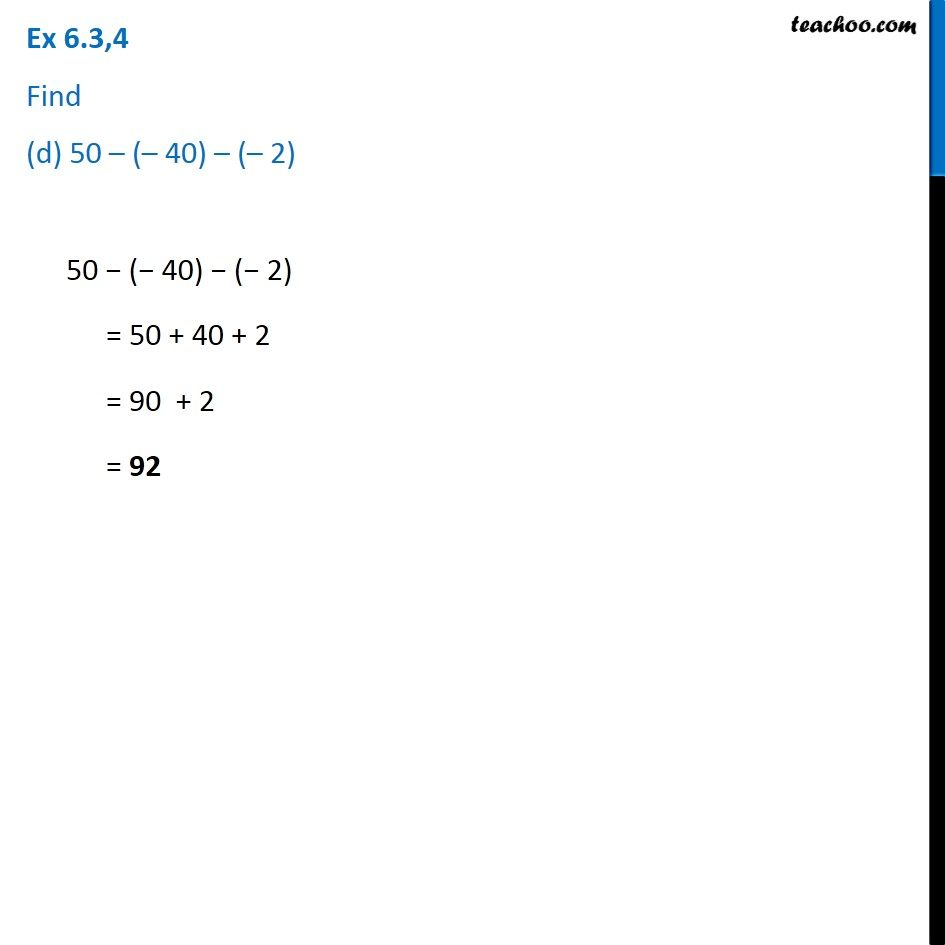1. Chapter 6 Class 6 Integers
2. Serial order wise
3. Ex 6.3

Transcript

Ex 6.3,4 Find (d) 50 – (– 40) – (– 2)50 − (− 40) − (− 2) = 50 + 40 + 2 = 90 + 2 = 92